# 协同效应视角下VC与创业企业的最优收益模型研究Research on the Optimal Income Model of VC and Entrepreneurial Enterprises from the Perspective of Synergy Effect

• 全文下载: PDF(1026KB)    PP.657-672   DOI: 10.12677/FIN.2019.96073
• 下载量: 156  浏览量: 200

Based on C-D production function and synergy effect between VC and entrepreneur, this article mainly discusses four differential game models in the continuous time, which is namely cooperative game model, non-cooperative one, VC-dominating Stackelberg model, entrepreneur-dominating Stackelberg model. HJB equation is used to find the optimal return strategy. It analyses the effect on different VC shareholding ratios to the optimal return and synergy incentives. Matlab 7.0 is used to draft the relationship between relative parameters and optimal return strategy. Sensitivity analysis of model parameters is performed. The results show that entrepreneurs are more inclined to provide synergy incentives and bring higher total return when VC shareholding ratio is less than 50%; while it is greater than 50%, VC is more inclined to provide synergy incentives and bring higher total return.

1. 引言

2. 模型相关说明

2.1. 模型相关变量Table 1. The main variables of the model

1. 模型主要变量

2.2. 模型相关假设

${H}^{\prime }=\delta {\left({e}_{V}{e}_{E}\right)}^{\frac{1}{2}}-\theta H,H\left(0\right)={H}_{0}\ge 0$

$\pi \left(t\right)=c{\left({e}_{V}{e}_{E}\right)}^{\frac{1}{2}}+d\cdot H\left(t\right)$

2.3. VC和创业企业协同的目标函数推导

$\pi \left(t\right)=c*{\left({e}_{V}{e}_{E}\right)}^{\frac{1}{2}}+d\cdot H\left(t\right)$

(1) VC提供协同效应激励的双方目标函数

${\pi }_{V}=\underset{0}{\overset{+\infty }{\int }}{\text{e}}^{-rt}\left\{\eta \left(t\right)\left[c{\left({e}_{V}{e}_{E}\right)}^{\frac{1}{2}}+d\cdot H\left(t\right)\right]-\frac{1}{2}{p}_{V}{e}_{V}^{2}-\tau \frac{1}{2}{p}_{E}{e}_{E}^{2}\right\}\text{d}t$

${\pi }_{E}=\underset{0}{\overset{+\infty }{\int }}{\text{e}}^{-rt}\left\{\left[1-\eta \left(t\right)\right]\left[c{\left({e}_{V}{e}_{E}\right)}^{\frac{1}{2}}+d\cdot H\left(t\right)\right]-\frac{1}{2}\left(1-\tau \right){p}_{E}{e}_{E}^{2}\right\}\text{d}t$

$\pi =\underset{0}{\overset{+\infty }{\int }}{\text{e}}^{-rt}\left\{\left[c{\left({e}_{V}{e}_{E}\right)}^{\frac{1}{2}}+d\cdot H\left(t\right)\right]-\frac{1}{2}{p}_{V}{e}_{V}^{2}-\frac{1}{2}{p}_{E}{e}_{E}^{2}\right\}\text{d}t$

(2) 创业企业提供协同效应激励的双方目标函数

${\pi }_{V}=\underset{0}{\overset{+\infty }{\int }}{\text{e}}^{-rt}\left\{\eta \left(t\right)\left[c{\left({e}_{V}{e}_{E}\right)}^{\frac{1}{2}}+d\cdot H\left(t\right)\right]-\frac{1}{2}\left(1-\rho \right){p}_{V}{e}_{V}^{2}\right\}\text{d}t$

${\pi }_{E}=\underset{0}{\overset{+\infty }{\int }}{\text{e}}^{-rt}\left\{\left[1-\eta \left(t\right)\right]\left[c{\left({e}_{V}{e}_{E}\right)}^{\frac{1}{2}}+d\cdot H\left(t\right)\right]-\frac{1}{2}{p}_{E}{e}_{E}^{2}-\frac{1}{2}\rho {p}_{V}{e}_{V}^{2}\right\}\text{d}t$

$\pi =\underset{0}{\overset{+\infty }{\int }}{\text{e}}^{-rt}\left\{\left[c{\left({e}_{V}{e}_{E}\right)}^{\frac{1}{2}}+d\cdot H\left(t\right)\right]-\frac{1}{2}{p}_{V}{e}_{V}^{2}-\frac{1}{2}{p}_{E}{e}_{E}^{2}\right\}\text{d}t$

3. 最优策略模型构建与求解

3.1. 完全信息条件下最优微分收益模型构建与求解

${V}^{*}\left(H\right)=\frac{d}{r+\theta }H+\frac{{\left[c\left(r+\theta \right)+\delta d\right]}^{2}}{4r{\left(r+\theta \right)}^{2}{p}_{V}^{\frac{1}{2}}{p}_{E}^{\frac{1}{2}}}$(1)

${\pi }_{E}=\underset{0}{\overset{+\infty }{\int }}{\text{e}}^{-rt}\left\{\left[c{\left({e}_{V}{e}_{E}\right)}^{\frac{1}{2}}+d\cdot H\left(t\right)\right]-\frac{1}{2}{p}_{V}{e}_{V}^{2}-\frac{1}{2}{p}_{E}{e}_{E}^{2}\right\}\text{d}t$

$r\cdot V\left(H\right)=\underset{\begin{array}{l}{e}_{V}\ge 0\\ {e}_{E}\ge 0\end{array}}{\mathrm{max}}\left\{\left[c{\left({e}_{V}{e}_{E}\right)}^{\frac{1}{2}}\right]+d\cdot H\left(t\right)-\frac{1}{2}{p}_{V}{e}_{V}^{2}-\frac{1}{2}{p}_{E}{e}_{E}^{2}+{V}^{\prime }\left(H\right)\left[\delta {\left({e}_{V}{e}_{E}\right)}^{\frac{1}{2}}-\theta H\right]\right\}$

${V}^{*}\left(H\right)=\frac{d}{r+\theta }H+\frac{{\left[c\left(r+\theta \right)+\delta d\right]}^{2}}{4r{\left(r+\theta \right)}^{2}{p}_{V}^{\frac{1}{2}}{p}_{E}^{\frac{1}{2}}}$

3.2. VC和创业企业不合作的最优微分收益模型构建与求解

${V}_{1}^{**}\left(H\right)=\frac{d\eta }{r+\theta }\cdot H+\frac{3{\eta }^{\frac{3}{2}}{\left(1-\eta \right)}^{\frac{1}{2}}{\left[c\left(r+\theta \right)+d\delta \right]}^{2}}{8r{\left(r+\theta \right)}^{2}{p}_{V}^{\frac{1}{2}}{p}_{E}^{\frac{1}{2}}}$(2)

${V}_{2}^{**}\left(H\right)=\frac{d\left(1-\eta \right)}{r+\theta }\cdot H+\frac{3{\eta }^{\frac{1}{2}}{\left(1-\eta \right)}^{\frac{3}{2}}{\left[c\left(r+\theta \right)+d\delta \right]}^{2}}{8r{\left(r+\theta \right)}^{2}{p}_{V}^{\frac{1}{2}}{p}_{E}^{\frac{1}{2}}}$(3)

${V}^{**}\left(H\right)=\frac{d}{r+\theta }\cdot H+\frac{3{\eta }^{\frac{1}{2}}{\left(1-\eta \right)}^{\frac{1}{2}}{\left[c\left(r+\theta \right)+d\delta \right]}^{2}}{8r{\left(r+\theta \right)}^{2}{p}_{V}^{\frac{1}{2}}{p}_{E}^{\frac{1}{2}}}$(4)

$r\cdot {V}_{1}\left(H\right)=\underset{\eta \ge 0}{\mathrm{max}}\left\{\eta \left[c\cdot {\left({e}_{V}{e}_{E}\right)}^{\frac{1}{2}}+d\cdot H\right]-\frac{1}{2}{p}_{V}{e}_{V}^{2}+{{V}^{\prime }}_{1}\left(H\right)\left[\delta \cdot {\left({e}_{V}{e}_{E}\right)}^{\frac{1}{2}}-\theta \cdot H\right]\right\}$

$S.T.:{e}_{V}\in \mathrm{arg}\mathrm{max}{V}_{1}\left(H\right),{e}_{E}\in \mathrm{arg}\mathrm{max}{V}_{2}\left(H\right)$(5)

$r\cdot {V}_{2}\left(H\right)=\underset{\eta \ge 0}{\mathrm{max}}\left\{\left(1-\eta \right)\left[c\cdot {\left({e}_{V}{e}_{E}\right)}^{\frac{1}{2}}+d\cdot H\right]-\frac{1}{2}{p}_{E}{e}_{E}^{2}+{{V}^{\prime }}_{2}\left(H\right)\left[\delta \cdot {\left({e}_{V}{e}_{E}\right)}^{\frac{1}{2}}-\theta \cdot H\right]\right\}$

$S.T.:{e}_{V}\in \mathrm{arg}\mathrm{max}{V}_{1}\left(H\right),{e}_{E}\in \mathrm{arg}\mathrm{max}{V}_{2}\left(H\right)$(6)

${e}_{V}=\frac{{\left[c\left(1-\eta \right)+\delta \cdot {{V}^{\prime }}_{2}\left(H\right)\right]}^{\frac{1}{4}}\cdot {\left[c\eta +\delta \cdot {{V}^{\prime }}_{1}\left(H\right)\right]}^{\frac{3}{4}}}{2{p}_{V}^{\frac{3}{4}}{p}_{E}^{\frac{1}{4}}}$(7)

${e}_{E}=\frac{{\left[c\left(1-\eta \right)+\delta \cdot {{V}^{\prime }}_{2}\left(H\right)\right]}^{\frac{3}{4}}\cdot {\left[c\eta +\delta \cdot {{V}^{\prime }}_{1}\left(H\right)\right]}^{\frac{1}{4}}}{2{p}_{V}^{\frac{1}{4}}{p}_{E}^{\frac{3}{4}}}$(8)

$r\cdot {{V}^{\prime }}_{1}\left(H\right)=\left[d\eta -\theta \cdot {{V}^{\prime }}_{1}\left(H\right)\right]\cdot H+\frac{3\cdot {\left[c\eta +\delta \cdot {{V}^{\prime }}_{1}\left(H\right)\right]}^{\frac{3}{2}}\cdot {\left[c\left(1-\eta \right)+\delta \cdot {{V}^{\prime }}_{2}\left(H\right)\right]}^{\frac{1}{2}}}{8{p}_{V}^{\frac{1}{2}}{p}_{E}^{\frac{1}{2}}}$(9)

$r\cdot {{V}^{\prime }}_{2}\left(H\right)=\left[d\left(1-\eta \right)-\theta \cdot {{V}^{\prime }}_{2}\left(H\right)\right]\cdot H+\frac{3\cdot {\left[c\eta +\delta \cdot {{V}^{\prime }}_{1}\left(H\right)\right]}^{\frac{1}{2}}\cdot {\left[c\left(1-\eta \right)+\delta \cdot {{V}^{\prime }}_{2}\left(H\right)\right]}^{\frac{3}{2}}}{{8}_{V}^{\frac{1}{2}}{p}_{E}^{\frac{1}{2}}}$(10)

${V}_{1}^{**}\left(H\right)=\frac{d\eta }{r+\theta }\cdot H+\frac{3{\eta }^{\frac{3}{2}}{\left(1-\eta \right)}^{\frac{1}{2}}{\left[c\left(r+\theta \right)+d\delta \right]}^{2}}{8r{\left(r+\theta \right)}^{2}{p}_{V}^{\frac{1}{2}}{p}_{E}^{\frac{1}{2}}}$

${V}_{2}^{**}\left(H\right)=\frac{d\left(1-\eta \right)}{r+\theta }\cdot H+\frac{3{\eta }^{\frac{1}{2}}{\left(1-\eta \right)}^{\frac{3}{2}}{\left[c\left(r+\theta \right)+d\delta \right]}^{2}}{8r{\left(r+\theta \right)}^{2}{p}_{V}^{\frac{1}{2}}{p}_{E}^{\frac{1}{2}}}$

${V}^{**}\left(H\right)=\frac{d}{r+\theta }\cdot H+\frac{3{\eta }^{\frac{1}{2}}{\left(1-\eta \right)}^{\frac{1}{2}}{\left[c\left(r+\theta \right)+d\delta \right]}^{2}}{8r{\left(r+\theta \right)}^{2}{p}_{V}^{\frac{1}{2}}{p}_{E}^{\frac{1}{2}}}$

3.3. 不完全信息条件下VC Stackelberg博弈模型建立与求解

${\tau }^{***}=\frac{7\eta -3}{1+3\eta }$，其中， $\frac{3}{7}<\eta <1$(11)

${V}_{1}^{***}\left(H\right)=\frac{d\eta }{r+\theta }\cdot H+\frac{{\left(1+3\eta \right)}^{2}{\left[c\left(r+\theta \right)+d\delta \right]}^{2}}{64r{\left(r+\theta \right)}^{2}{p}_{V}^{\frac{1}{2}}{p}_{E}^{\frac{1}{2}}}$(12)

${V}_{2}^{***}\left(H\right)=\frac{d\left(1-\eta \right)}{r+\theta }\cdot H+\frac{3\left(1-\eta \right)\left(1+3\eta \right){\left[c\left(r+\theta \right)+d\delta \right]}^{2}}{32r{\left(r+\theta \right)}^{2}{p}_{V}^{\frac{1}{2}}{p}_{E}^{\frac{1}{2}}}$(13)

${V}^{***}\left(H\right)=\frac{d}{r+\theta }\cdot H+\frac{\left(7-3\eta \right)\left(1+3\eta \right){\left[c\left(r+\theta \right)+d\delta \right]}^{2}}{64r{\left(r+\theta \right)}^{2}{p}_{V}^{\frac{1}{2}}{p}_{E}^{\frac{1}{2}}}$(14)

$r\cdot {V}_{2}\left(H\right)=\underset{{e}_{E}\ge 0}{\mathrm{max}}\left\{\left(1-\eta \right)\left[c\cdot {\left({e}_{V}{e}_{E}\right)}^{\frac{1}{2}}+d\cdot H\right]-\frac{1}{2}\left(1-\tau \right){p}_{E}{e}_{E}^{2}+{{V}^{\prime }}_{2}\left(H\right)\left[\delta \cdot {\left({e}_{V}{e}_{E}\right)}^{\frac{1}{2}}-\theta \cdot H\right]\right\}$(15)

(15)式对 ${e}_{E}$ 求一阶导数，并令其等于零，可以求得：

${e}_{E}=\frac{{e}_{V}^{\frac{1}{3}}{\left[\delta \cdot {{V}^{\prime }}_{2}\left(H\right)-c\eta +c\right]}^{\frac{2}{3}}}{{4}^{\frac{1}{3}}{p}_{E}^{\frac{2}{3}}{\left(1-\tau \right)}^{\frac{2}{3}}}$(16)

$r\cdot {V}_{1}\left(H\right)=\underset{\begin{array}{c}{e}_{V}\ge 0\\ 0<\tau <1\end{array}}{\mathrm{max}}\left\{\eta \left[c\cdot {\left({e}_{V}{e}_{E}\right)}^{\frac{1}{2}}+d\cdot H\right]-\frac{1}{2}{p}_{V}{e}_{V}^{2}-\frac{1}{2}\tau {p}_{E}{e}_{E}^{2}+{V}_{1}{}^{\prime }\left(H\right)\left[\delta \cdot {\left({e}_{V}{e}_{E}\right)}^{\frac{1}{2}}-\theta \cdot H\right]\right\}$(17)

${e}_{V}^{***}=\frac{\left(1+3\eta \right)\left[c\left(r+\theta \right)+d\delta \right]}{8\left(r+\theta \right){p}_{V}^{\frac{3}{4}}{p}_{E}^{\frac{1}{4}}}$

${e}_{E}^{***}=\frac{\left(1+3\eta \right)\left[c\left(r+\theta \right)+d\delta \right]}{8\left(r+\theta \right){p}_{V}^{\frac{1}{4}}{p}_{E}^{\frac{3}{4}}}$

${\tau }^{***}=\frac{7\eta -3}{1+3\eta }$，其中， $\frac{3}{7}<\eta <1$

${V}_{1}^{***}\left(H\right)=\frac{d\eta }{r+\theta }\cdot H+\frac{{\left(1+3\eta \right)}^{2}{\left[c\left(r+\theta \right)+d\delta \right]}^{2}}{64r{\left(r+\theta \right)}^{2}{p}_{V}^{\frac{1}{2}}{p}_{E}^{\frac{1}{2}}}$

${V}_{2}^{***}\left(H\right)=\frac{d\left(1-\eta \right)}{r+\theta }\cdot H+\frac{3\left(1-\eta \right)\left(1+3\eta \right){\left[c\left(r+\theta \right)+d\delta \right]}^{2}}{32r{\left(r+\theta \right)}^{2}{p}_{V}^{\frac{1}{2}}{p}_{E}^{\frac{1}{2}}}$

${V}^{***}\left(H\right)=\frac{d}{r+\theta }\cdot H+\frac{\left(7-3\eta \right)\left(1+3\eta \right){\left[c\left(r+\theta \right)+d\delta \right]}^{2}}{64r{\left(r+\theta \right)}^{2}{p}_{V}^{\frac{1}{2}}{p}_{E}^{\frac{1}{2}}}$

3.4. 不完全信息条件下创业企业主导型Stackelberg博弈模型建立与求解

${\rho }^{****}=\frac{4-7\eta }{4-3\eta }$，其中， $0<\eta <\frac{4}{7}$(18)

${V}_{1}^{****}\left(H\right)=\frac{d\eta }{r+\theta }\cdot H+\frac{3\eta \left(4-3\eta \right){\left[c\left(r+\theta \right)+\delta d\right]}^{2}}{32r{\left(r+\theta \right)}^{2}{p}_{V}^{\frac{1}{2}}{p}_{E}^{\frac{1}{2}}}$(19)

${V}_{2}^{****}\left(H\right)=\frac{d\left(1-\eta \right)}{r+\theta }\cdot H+\frac{{\left(4-3\eta \right)}^{2}\cdot {\left[c\left(r+\theta \right)+d\delta \right]}^{2}}{64r{\left(r+\theta \right)}^{2}{p}_{V}^{\frac{1}{2}}{p}_{E}^{\frac{1}{2}}}$(20)

${V}^{****}\left(H\right)=\frac{d}{r+\theta }\cdot H+\frac{\left(4-3\eta \right)\left(4+3\eta \right)\cdot {\left[c\left(r+\theta \right)+d\delta \right]}^{2}}{64r{\left(r+\theta \right)}^{2}{p}_{V}^{\frac{1}{2}}{p}_{E}^{\frac{1}{2}}}$(21)

$r\cdot {V}_{1}\left(H\right)=\underset{\begin{array}{l}{e}_{V}\ge 0\\ 0<\tau <1\end{array}}{\mathrm{max}}\left\{\eta \left[c\cdot {\left({e}_{V}{e}_{E}\right)}^{\frac{1}{2}}+d\cdot H\right]-\frac{1}{2}\left(1-\rho \right){p}_{V}{e}_{V}^{2}+{{V}^{\prime }}_{1}\left(H\right)\left[\delta \cdot {\left({e}_{V}{e}_{E}\right)}^{\frac{1}{2}}-\theta \cdot H\right]\right\}$(22)

${e}_{V}^{****}=\frac{\left(4-3\eta \right)\left[c\left(r+\theta \right)+d\delta \right]}{8\left(r+\theta \right){p}_{V}^{\frac{3}{4}}{p}_{E}^{\frac{1}{4}}}$

${e}_{E}^{****}=\frac{\left(4-3\eta \right)\left[c\left(r+\theta \right)+d\delta \right]}{8\left(r+\theta \right){p}_{V}^{\frac{1}{4}}{p}_{E}^{\frac{3}{4}}}$

${\rho }_{1}^{****}=\frac{4-7\eta }{4-3\eta }$，其中， $0<\eta <\frac{4}{7}$

${V}_{1}^{****}\left(H\right)=\frac{d\eta }{r+\theta }\cdot H+\frac{3\eta \left(4-3\eta \right){\left[c\left(r+\theta \right)+\delta d\right]}^{2}}{32r{\left(r+\theta \right)}^{2}{p}_{V}^{\frac{1}{2}}{p}_{E}^{\frac{1}{2}}}$

${V}_{2}^{****}\left(H\right)=\frac{d\left(1-\eta \right)}{r+\theta }\cdot H+\frac{{\left(4-3\eta \right)}^{2}\cdot {\left[c\left(r+\theta \right)+d\delta \right]}^{2}}{64r{\left(r+\theta \right)}^{2}{p}_{V}^{\frac{1}{2}}{p}_{E}^{\frac{1}{2}}}$

${V}^{****}\left(H\right)=\frac{d}{r+\theta }\cdot H+\frac{\left(4-3\eta \right)\left(4+3\eta \right)\cdot {\left[c\left(r+\theta \right)+d\delta \right]}^{2}}{64r{\left(r+\theta \right)}^{2}{p}_{V}^{\frac{1}{2}}{p}_{E}^{\frac{1}{2}}}$

4. 博弈均衡结果比较分析

(1)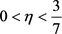时，

① 创业投资企业的最优利润排序为：

${V}_{1}^{**}\left(H\right)<{V}_{1}^{****}\left(H\right)$

② 创业企业的最优利润排序如下：

${V}_{2}^{**}\left(H\right)<{V}_{2}^{****}\left(H\right)$

③ 整个创业链的最优利润排序如下：

${V}^{**}\left(H\right)<{V}^{****}\left(H\right)<{V}^{*}\left(H\right)$

(2) $\frac{3}{7}<\eta <\frac{4}{7}$ 时，

① 创业投资企业的最优利润排序为：

${V}_{1}^{**}\left(H\right)<{V}_{1}^{***}\left(H\right)<{V}_{1}^{****}\left(H\right)$

② 创业企业的最优利润排序如下：

${V}_{2}^{**}\left(H\right)<{V}_{2}^{****}\left(H\right)<{V}_{2}^{***}\left(H\right)$

③ 整个创业链的最优利润排序如下：

$\eta \in \left(\frac{3}{7},\frac{1}{2}\right)$ 时， ${V}^{**}\left(H\right)<{V}^{***}\left(H\right)<{V}^{****}\left(H\right)<{V}^{*}\left(H\right)$

$\eta \in \left(\frac{1}{2},\frac{4}{7}\right)$ 时， ${V}^{**}\left(H\right)<{V}^{****}\left(H\right)<{V}^{***}\left(H\right)<{V}^{*}\left(H\right)$

(3) $\frac{4}{7}<\eta <1$ 时，

① 创业投资企业的最优利润排序为：

${V}_{1}^{**}\left(H\right)<{V}_{1}^{***}\left(H\right)$

② 创业企业的最优利润排序如下：

${V}_{2}^{**}\left(H\right)<{V}_{2}^{***}\left(H\right)$

③ 整个创业链的最优利润排序如下：

${V}^{**}\left(H\right)<{V}^{***}\left(H\right)<{V}^{*}\left(H\right)$

$0<\eta <\frac{3}{7}$ 时，

${V}_{1}^{**}\left(H\right)-{V}_{1}^{****}\left(H\right)=\frac{3\eta \left[4{\eta }^{\frac{1}{2}}{\left(1-\eta \right)}^{\frac{1}{2}}-\left(4-3\eta \right)\right]\cdot {\left[c\left(r+\theta \right)+d\delta \right]}^{2}}{32r{\left(r+\theta \right)}^{2}{p}_{V}^{\frac{1}{2}}{p}_{E}^{\frac{1}{2}}}<0$

5. 数值仿真分析

${V}^{*}\left(H\right)=5H+86.62$ ；VC与创业企业非合作情形下，协同效应 ${H}_{t=10}^{**}=15.64*{\eta }^{\frac{1}{2}}{\left(\text{1}-\eta \right)}^{\frac{1}{2}}$，创业链最优总收益为 ${V}^{**}\left(H\right)=5H+129.93*{\eta }^{\frac{1}{2}}{\left(\text{1}-\eta \right)}^{\frac{1}{2}}$，创业投资企业的最优收益为 ${V}_{1}^{**}\left(H\right)=5\eta H+129.93*{\eta }^{\frac{3}{2}}{\left(1-\eta \right)}^{\frac{1}{2}}$，创业企业的最优收益为 ${V}_{2}^{**}\left(H\right)=5\left(1-\eta \right)H+129.93*{\eta }^{\frac{1}{2}}{\left(1-\eta \right)}^{\frac{3}{2}}$ ；VC主导型主从博弈情形下，协同效应

${H}_{t=10}^{***}=3.91*\left(1+3\eta \right)$，创业链最优总收益为 ${V}^{***}\left(H\right)=5H+5.41*\left(7-3\eta \right)\left(1+3\eta \right)$，创业投资企业的最优收益为 ${V}_{1}^{***}\left(H\right)=5\eta H+5.41*{\left(1+3\eta \right)}^{2}$，创业企业的最优收益为 ${V}_{2}^{***}\left(H\right)=5\left(1-\eta \right)H+32.48*\left(1-\eta \right)\left(1+3\eta \right)$ ；创业企业主导型主从博弈情形下，协同效应 ${H}_{t=10}^{****}=0.39*\left(4-3\eta \right)$，创业链最优总收益为 ${V}^{***}\left(H\right)=5H+$ $5.41*\left(4-3\eta \right)\left(4+3\eta \right)$，创业投资企业的最优收益为 ${V}_{1}^{***}\left(H\right)=5\eta H+32.48*\eta \left(4-3\eta \right)$，创业企业的最优收益为 ${V}_{2}^{***}\left(H\right)=5\left(1-\eta \right)H+5.41*{\left(4-3\eta \right)}^{2}$

5.1. 不同博弈情形下VC持股比例与最优收益的对比分析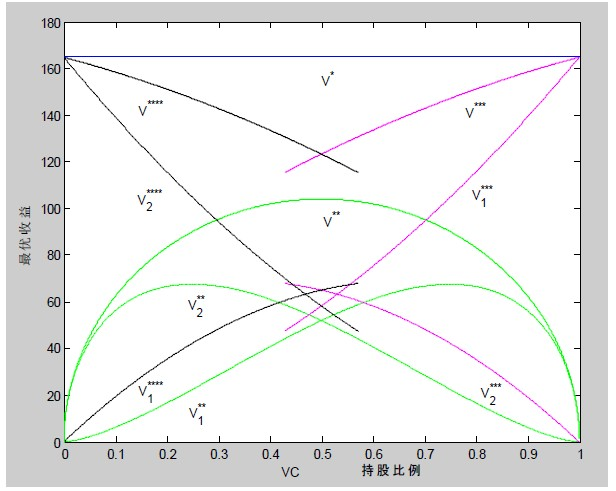Figure 1. The comparative analysis of VC shareholding ratio and optimal returns in different game situations when t = 10

1. t = 10时，不同博弈情形下VC持股比例与最优收益的对比分析

$\eta \in \left[\frac{3}{7},\frac{1}{2}\right]$，由创业企业为创业投资企业提供协同效应“激励因子”；而VC持股比例 $\eta \in \left[\frac{1}{2},\frac{4}{7}\right]$，由创

5.2. 持股比例对最优激励因子的影响分析

(1) 在不完全信息条件下由创业投资主导型的Stackelberg博弈均衡时，创业投资企业提供给创业企业的最优激励因子为：

${\tau }^{***}=\frac{7\eta -3}{1+3\eta }$，其中， $\frac{3}{7}<\eta <1$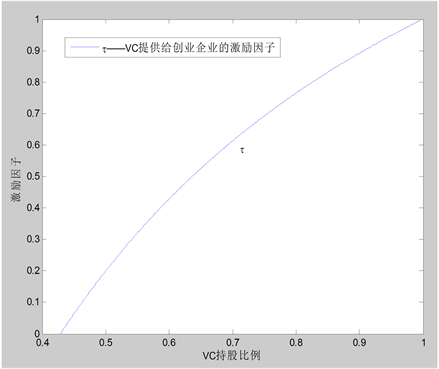Figure 2. The relationship between the optimal incentive factor and VC shareholding ratio in VC-dominated master-slave game

2. VC主导型主从博弈下最优激励因子与VC持股比例的关系

(2) 在不完全信息条件下由创业企业主导型的Stackelberg博弈均衡时，创业企业提供给创业投资企业的最优激励因子为：

${\rho }^{****}=\frac{4-7\eta }{4-3\eta }$，其中， $0<\eta <\frac{4}{7}$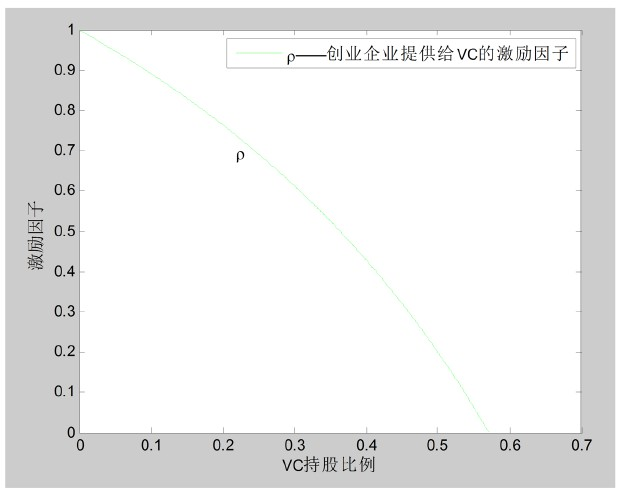Figure 3. The relationship between the optimal incentive factor and VC shareholding ratio in the entrepreneurial enterprise-dominated master-slave game

3. 创业企业主导型主从博弈下最优激励因子与VC持股比例的关系

(3) $\frac{3}{7}<\eta <\frac{4}{7}$ 时，VC与创业企业激励因子的对比分析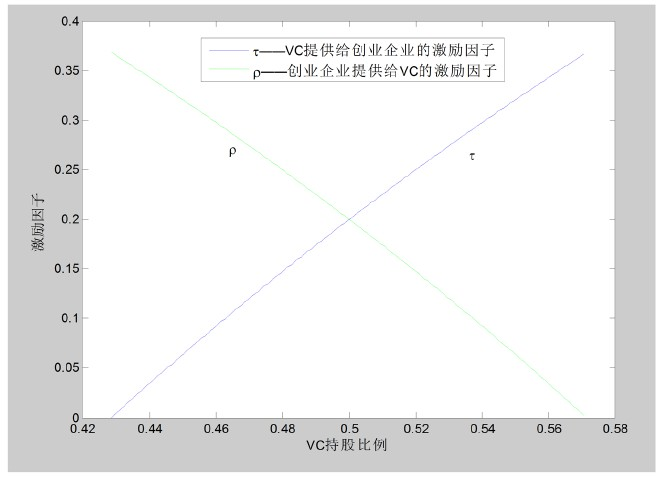Figure 4. The relationship between the optimal incentive factor and VC shareholding ratio when $\frac{3}{7}<\eta <\frac{4}{7}$

4. $\frac{3}{7}<\eta <\frac{4}{7}$ 时最优激励因子与VC持股比例的关系

6. 结论

  张帏, 姜彦福, 陈耀刚. 风险投资中的代理问题、风险分担与制度安排[J]. 科研管理, 2002, 23(1): 122-127.  王茵田, 黄张凯, 陈梦. “不平等条约？”: 我国对赌协议的风险因素分析[J]. 金融研究, 2017(8): 117-128.  金永红, 奚玉芹, 叶中行. 风险投资中的逆向选择: 分离均衡式契约安排[J]. 系统工程学报, 2002, 17(6): 556-561.  王雁茜, 周启功. 风险投资投资过程的逆向选择和道德风险[J]. 科研管理, 2002, 23(3): 105-111.  Elitzur, R. and Gavious, A. (2003) A Multi-Period Game Theoretic Model of Venture Capitalists and Entrepreneurs. European Journal of Operational Research, 144, 440-453. https://doi.org/10.1016/S0377-2217(02)00144-3  Reid, J.D. (1977) The Theory of Share Tenancy Revisited-again. Journal of Political Economy, 85, 403-407. https://doi.org/10.1086/260571  Casamatta, C. (2003) Financing and Advising: Optimal Financial Contracts with Venture Capitalists. The Journal of Finance, 58, 2059-2086. https://doi.org/10.1111/1540-6261.00597  Hellmann, T. (2006) IPOs, Acquisition and the Use of Convertible Securities in Venture Capital. Journal of Financial Economics, 81, 649-679. https://doi.org/10.1016/j.jfineco.2005.06.007  吴萌. 风险企业家考虑公平关切的风险投资多阶段激励模型[J]. 管理工程学报, 2016, 30(3): 72-80.  Geronikolaou, G. and Papachristou, G. (2016) Investor Competition and Project Risk in Venture Capital Investments. Economics Letters, 141, 67-69. https://doi.org/10.1016/j.econlet.2016.02.007  薛力, 郭菊娥. 风险投资机构内部融资模式对风险投资家股权投资策略的影响[J]. 系统管理学报, 2018, 27(2): 201-207.  Gorman, M. and Sahlman, W.A. (1989) What Do Venture Capitals Do? Journal of Business Venturing, 4, 231-248. https://doi.org/10.1016/0883-9026(89)90014-1  党兴华, 权小锋, 杨敏利. 风险投资委托代理关系中的监控与激励博弈分析[J]. 科研管理, 2007, 28(1): 155-160.  池仁勇. 区域中小企业创新网络的结点联结及其效率评价研究[J]. 管理世界, 2007(1): 105-112.  Fairchild, R. (2011) An Entrepreneur’s Choice of Venture Capitalist or Angel Financing: A Behavioral Game-Theoretic Approach. Journal of Business Venturing, 26, 359-374. https://doi.org/10.1016/j.jbusvent.2009.09.003  Vergara, M., Bonilla, C.A. and Sepulveda, J.P. (2016) The Com-plementarity Effect: Effort and Sharing in the Entrepreneur and Venture Capital Contract. European Journal of Operational Research, 254, 1017-1025. https://doi.org/10.1016/j.ejor.2016.04.040  赵黎明. 基于创业链声誉的风险投资与风险企业合作的微分对策模型[J]. 管理工程学报, 2016, 30(1): 169-175.